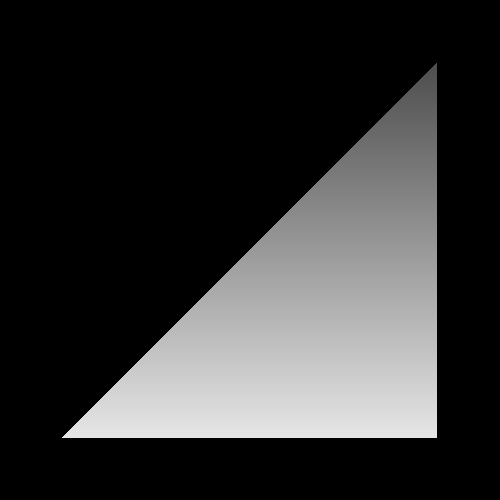## Chapter 2. Playing with Colors

Table of Contents

Fragment Position Display
Vertex Attributes
In Review
Glossary

This tutorial will show how to provide some color to the triangle from the previous tutorial. Instead of just giving the triangle a solid color, we will use two methods to provide it with varying color across its surface. The first method will use the fragment's position to compute a color, while the second will use per-vertex data to compute a color.

## Fragment Position Display

As we stated in the overview, part of the fragment's data includes the position of the fragment on the screen. Thus, if we want to vary the color of a triangle across its surface, We can access this data in our fragment shader and use it to compute the final color for that fragment. This is done in the Fragment Position tutorial, who's main file is FragPosition.cpp.

In this tutorial, and all future ones, shaders will be loaded from files instead of hard-coded strings in the .cpp file. To support this, the framework has the Framework::LoadShader and Framework::CreateProgram functions. These work similarly to the previous tutorial's CreateShader and CreateProgram, except that LoadShader takes a filename instead of a shader file.

The FragPosition tutorial loads two shaders, the vertex shader data/FragPosition.vert and the fragment shader data/FragPosition.frag. The vertex shader is identical to the one in the last tutorial. The fragment shader is very new, however:

Example 2.1. FragPosition's Fragment Shader

#version 330

out vec4 outputColor;

void main()
{
float lerpValue = gl_FragCoord.y / 500.0f;

outputColor = mix(vec4(1.0f, 1.0f, 1.0f, 1.0f),
vec4(0.2f, 0.2f, 0.2f, 1.0f), lerpValue);
}

gl_FragCoord is a built-in variable that is only available in a fragment shader. It is a vec3, so it has an X, Y, and Z component. The X and Y values are in window coordinates, so the absolute value of these numbers will change based on the window's resolution. Recall that window coordinates put the origin at the bottom-left corner. So fragments along the bottom of the triangle would have a lower Y value than those at the top.

The idea with this shader is that the color of a fragment will be based on the Y value of its window position. The 500.0f is the height of the window (unless you resize the window). The division in the first line of the function simply converts the Y position to the [0, 1] range, where 1 is at the top of the window and 0 is at the bottom.

The second line uses this [0, 1] value to perform a linear interpolation between two colors. The mix function is one of the many, many standard functions that the OpenGL Shading Language provides. Many of these functions, like mix, are vectorized. That is, some of their parameters can be vectors, and when they are, they will perform their operations on each component of the vector simultaneously. In this case, the dimensionality of the first two parameters must match.

The mix function performs a linear interpolation. It will return exactly the first parameter if the third parameter is 0, and it will return exactly the second parameter if the third parameter is 1. If the third parameter is between 0 and 1, it will return a value between the two other parameters, based on the third parameter.

### Note

The third parameter to mix must be on the range [0, 1]. However, GLSL will not check this or do the clamping for you. If it is not on this range, the result of the mix function will be undefined. Undefined is the OpenGL shorthand for, I do not know, but it is probably not what you want.

We get the following image:

Figure 2.1. Fragment PositionIn this case, the bottom of the triangle, the one closest to a Y of 0, will be the most white. While the top of the triangle, the parts closest to a Y of 500, will have the darkest color.

Other than the fragment shader, nothing much changes in the code.

Fork me on GitHub